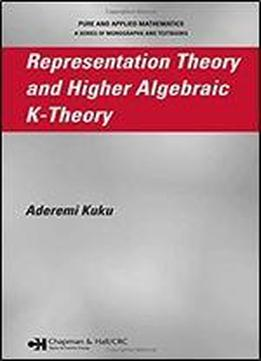# Representation Theory And Higher Algebraic K-theory (pure & Applied Mathematics) by Aderemi Kuku / 2006 / English / PDF

Representation Theory and Higher Algebraic K-Theory is the first book to present higher algebraic K-theory of orders and group rings as well as characterize higher algebraic K-theory as Mackey functors that lead to equivariant higher algebraic K-theory and their relative generalizations. Thus, this book makes computations of higher K-theory of group rings more accessible and provides novel techniques for the computations of higher K-theory of finite and some infinite groups. Authored by a premier authority in the field, the book begins with a careful review of classical K-theory, including clear definitions, examples, and important classical results. Emphasizing the practical value of the usually abstract topological constructions, the author systematically discusses higher algebraic K-theory of exact, symmetric monoidal, and Waldhausen categories with applications to orders and group rings and proves numerous results. He also defines profinite higher K- and G-theory of exact categories, orders, and group rings. Providing new insights into classical results and opening avenues for further applications, the book then uses representation-theoretic techniques-especially induction theory-to examine equivariant higher algebraic K-theory, their relative generalizations, and equivariant homology theories for discrete group actions. The final chapter unifies Farrell and Baum-Connes isomorphism conjectures through Davis-Luck assembly maps.

views: 268